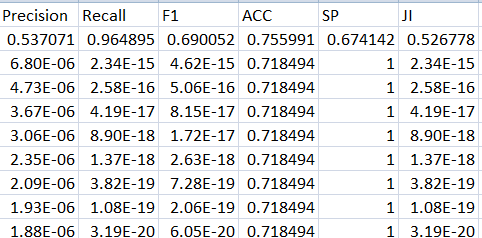# Precision,recall and f1 score values in EXP

I am using doing binary segmentation using Signet, IoU loss. the problem is Precision, recall and f1 score values become exp. kindly guide me if there is an issue with my loss function or there is any other issue.i have pasted the loss code below
Thank you

``````
class IoULoss(nn.Module):
def __init__(self, weight=None, size_average=True):
super(IoULoss, self).__init__()

def forward(self, inputs, targets, smooth=1):

inputs = inputs.view(-1)
targets = targets.view(-1)

intersection = (inputs * targets).sum()
total = (inputs + targets).sum()
union = total - intersection

IoU = (intersection + smooth)/(union + smooth)

return 1 - IoU
``````The result table indicates that the precision, recall, F1, and Ji are all zero. You can ignore the small numbers and basically treat them as zero values, so you should check your metric calculation as well as the results of your model.

Thank you @ptrblck for your response. here is the code for metric calculation.

``````def metric_calculation(input, target):
num_in_target = input.size(0)
y_pred = input.view(-1)
y_true = target.view(-1)
tp = (y_true * y_pred).sum(dim=0).to(torch.float32)
tn = ((1 - y_true) * (1 - y_pred)).sum(dim=0).to(torch.float32)
fp = ((1 - y_true) * y_pred).sum(dim=0).to(torch.float32)
fn = (y_true * (1 - y_pred)).sum(dim=0).to(torch.float32)
return tp,tn,fp,fn
``````

I have changed the model to Unet after some epoch, same error occurred.
I need your views, i have checked my data,it does not contain any nan value and data values are normalized between 0 and 1.So there is nothing wrong with data or model then what can be the issue. Any help will be appreciated

If I stop and then resume the training everything works fine. I have used PyTorch anomaly detection and even tried to clip gradient between -5 to 5 but it’s also not working. but the problem is what can be the issue then

1. Data does not contain any nan value.
2. Changing the model does not work.
3. Gradient clipping does not work.
4. learning rate is 1e^-3 at the start of training and updating it using lr scheduler
5. Apparently there is no issue in metric and loss calculation code.

then what can be the issue
kindly guide me

Any help will be appreciated
Thanks

I don’t see any NaNs in your output, so I’m unsure why you are checking for these invalid values.
In your table the scientific notation is used, which is indicating values close to zero.